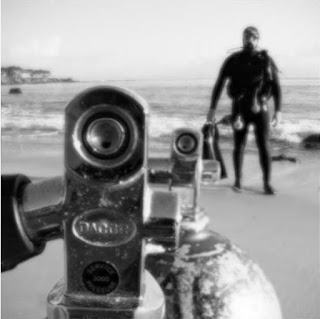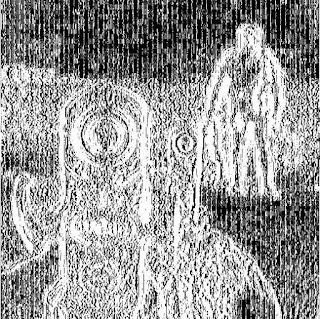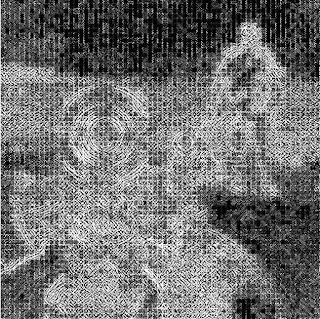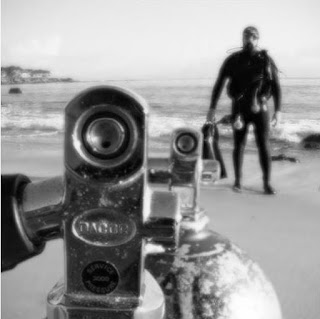Multi-Focus Image Fusion Technique using Stationary Wavelet Transform (SWT) | Multi-Focus Image Fusion Matlab Code | Image Fusion Matlab Code. The techniques of the multi-focus image fusion play an important role as an application in the field of Visual Sensor Network. Image fusion is one of the most demanding research areas in the field of image processing.

The wavelet transforms are widely used in this area. Image fusion using the wavelet transform provides great results. They are implemented using average and maximum rule operations. Usually, these techniques are applied to two input images i.e. left and right focused images which are very common practice. Here we will learn to apply the Image Fusion Technique using Stationary Wavelet Transform on three input multi-focused image datasets.

### Line by line explanation of image fusion technique using SWT on three input multi-focused image datasets (simple copy this code in the Matlab Editor and RUN)

% Clear command window
clc;

% Removes all variables from the workspace.
clear all;

% Deletes all figures including those with hidden handles.
close all;

% Read first input image and pre-process and store it in b
b = rgb2gray(a);

% Read second input image and pre-processand store it in d
d = rgb2gray(c);

% Read third input image and pre-processand store it in f
f = rgb2gray(e);

% Apply 1-level 2D-SWT on 'b' using 'sym2' wavelet family. It generates four components i.e. A1, H1, V1, D1. [A1 = Approximate, H1 = Horizontal, V1 = Vertical, D1 = Diagonal]
[A1, H1, V1, D1] = swt2(b, 1, 'sym2');

% Apply 1-level 2D-SWT on 'd' using 'sym2' wavelet family. It generates four components i.e. A2, H2, V2, D2. [A2 = Approximate, H2 = Horizontal, V2 = Vertical, D2 = Diagonal]
[A2, H2, V2, D2] = swt2(d, 1, 'sym2');

% Apply 1-level 2D-SWT on 'f' using 'sym2' wavelet family. It generates four components i.e. A3, H3, V3, D3. [A3 = Approximate, H3 = Horizontal, V3 = Vertical, D3 = Diagonal]
[A3, H3, V3, D3] = swt2(f, 1, 'sym2');

% Fusing approximate components using the average operation
% Here fusion is performed on the approximate components by taking the average of A1, A2, and A3 and storing it in A.
A = (A1 + A2 + A3)./3;

% Fusing horizontal components using the maximum operation. Here we try to find the best horizontal component out of H1, H2 and, H3.
% Maximum of H1 and H2 is taken and stored in H4.
H4 = max(H1,H2);

% Maximum of H2 and H3 is taken and stored in H5.
H5 = max(H2,H3);

% Maximum of H4 and H5 is taken and stored in H.
H = max(H4,H5);

% Fusing vertical components using the maximum operation. Here we try to find the best vertical component out of V1, V2 and, V3.
% Maximum of V1 and V2 is taken and stored in V4.
V4 = max(V1,V2);

% Maximum of V2 and V3 is taken and stored in V5.
V5 = max(V2,V3);

% Maximum of V4 and V5 is taken and stored in V.
V = max(V4,V5);

% Fusing diagonal components using the maximum operation. Here we try to find the best diagonal component out of D1, D2 and, D3.
% Maximum of D1 and D2 is taken and stored in D4.
D4 = max(D1,D2);

% Maximum of D2 and D3 is taken and stored in D5.
D5 = max(D2,D3);

% Maximum of D4 and D5 is taken and stored in D.
D = max(D4,D5);

% After the above steps A, H, V, and D hold the fused approximate and detailed components.
% Display approximate component.
figure;
imshow(A,[]);

% Display horizontal component.
figure;
imshow(H)

% Display vertical component.
figure;
imshow(V)

% Display diagonal component.
figure;
imshow(D)

% Apply inverse SWT on A, H, V, and D using 'sym2' wavelet family to get the final fused image i.e. 'imf'.
imf = iswt2(A, H, V, D, 'sym2');

% Display the final fused image.
figure;
imshow(imf,[]);

### Results of Image Fusion Technique using Stationary Wavelet TransformApproximate componentHorizontal componentVertical componentDiagonal componentFinal fused image

For more topics refer: https://www.gofastresearch.com/
Multi-Focus Image Fusion Technique using Stationary Wavelet Transform Matlab Code | Multi-Focus Image Fusion Matlab Code | Image Fusion Matlab CodeReviewed by IPR on May 29, 2020 Rating: 5